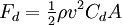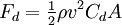# Energy used during vehicle trips from GPS data

• tgrandahl
In summary, Tyler Grandahl found an urge to understand how much energy is used while driving, and has started working on a GPX file to track energy used during a trip. He used 710171 J in acceleration, 1614412.8 J in fighting normal force, and 17182665 J in fighting drag. He wanted to have some control over the final energy calculation, so he is hoping to find an error in his current calculations. He also has started working on a worksheet to estimate the amount of energy used during a drive, and has attached it to this message.f

#### tgrandahl

Hello,

I have recently found an urge to understand the amount of energy that I use while driving.

I would like to be able to record tracking data via GPS in a GPX format, and then calculate the amount of energy that would be used to perform the same trip using various vehicle models.

I would also like to be able to realize the energy that may be rescued using regenerative braking in an electric vehicle or hybrid.

I have started working on this in an excel worksheet because I feel it will be easiest to layout the math necessary to estimate the energy used. The worksheet currently calculates force for acceleration, normal force, and drag.
Over my 7.1 mile journey I used:

710171 J accelerating,
1614412.8 J fighting normal force,
17182665 J fighting drag... Seriousley ?...

I wanted to have some control value to compare my calculations with to know if I am on track or not.

~7.1 mile trip,
~23 MPG,
~132x10^6 J / gallon of gasoline,
~12% of consumed energy reaches the wheels of my vehicle

(7.1/23)*132x10^6*0.12 = 48.9x10^4 J

My worksheet currently calculates 19.5x10^5 J being used

Although my vehicle is consuming roughly 40.7x10^5 J in gasoline during the drive my vehicle would have to be 50% efficient!

On top of that I am currently being very generous and not summing the energy used while going downhill even for the drag. Looking at the numbers it seems as if my error is in my drag calculation.W=FxD

I am using the typical drag coefficient and p values gamesguru listed here:

The actual weight of my vehicle, 1584Kg.

I do understand the resolution of my GPS data is rather poor. It was collected using my iphone during a quick trip. I need allot more points and more accurate altitude. However I did expect to be able to get some sensible results from it.

I wouldn't say physics is one of my real strengths so I am hoping this is a simple error. Can anyone here show me where I have steered off course? I have attached the worksheet, thank you for any help you can provide.

~Tyler Grandahl

#### Attachments

• energy calcs.xls
42.5 KB · Views: 177
Last edited by a moderator:
Ok, so maybe the question I should have asked is, am I taking the right approach to this?

I can type up the math that i am using in the worksheet if it would be helpful.

Is the approach i am taking to estimate the amount of work my car is doing during the trip valid?

I would like to clarify what is currently going on in the worksheet:

The trip calculations section shows extended trip data computed from the GPX input data:

-Delta T(s) calculates the elapsed time from the previous data point.

-Delta V is the change in velocity from the previous point. =Vcurrent-Vprevious

-Accel is the acceleration that was needed to reach the current velocity. =(Delta V)/(Delta T)

-Ascend/Descend compares the current altitude to previous altitude and returns a +1 for ascending and -1 for descending. This can be used as a multiplier in later calculations.

-Accel/Decel is the same idea as Ascend/Descend but with acceleration.

-Distance M 2D is the distance between the current gps coordinates and the previous gps coordinates computed in meters using the Haversine formula:

d = acos(sin(lat1).sin(lat2)+cos(lat1).cos(lat2).cos(long2−long1)).R

-Distance M 3D takes into account the change in altitude, calculating distance as the hypotenuse of a right triangle. distance M 2D as the base and the change in altitude as the height. D=SQRT((Distance 2D^2)+((Current Alt-Prev Alt)^2))

-Angle Rad is the angle of elevation of the road surface. computed from the 3D distance and change in elevation. =ASIN(ABS(current alt-previous alt)/3D distance)

Normal Force calculations:

-Normal Force (N) Calculates the normal force pulling the vehicle down the incline from the angle of elevation, and vehicle mass. =(9.8*mass)*SIN(Angle Elev)*"Ascend/Decend"

-Normal Force work (J) the work done over the 3D distance traveled. =Normal Force(N)*Distance M 3D

-Filtered brings over all the positive Normal Force work (J) values as i am interested in the net work done by the engine and not the breaks, these values are summed at the bottom of the column.

Acceleration:

-Accel force (N) is the force necessary to accelerate the vehicle, F=M*A

-Accel work (J) is the work done over the 3D distance traveled to accelerate the vehicle.

=Accel force*Distance M 3D

-Filtered is again only the positive values to be summed.

Drag: (the troublemaker)

-Drag Force (N) is calculated from the average of the current velocity and previous velocity, as well as generic values of p (air density), Ca (drag coef), and A (ref area). These user defined constants are entered in the top left corner of the worksheet.=0.5*p*drag*(((Vcurrent+Vprevious)/2)^2)

-Drag Work(J) calculates the work needed to overcome the drag force over the 3D distance traveled.

-W=Drag Force*Distance 3D

-Filtered only brings over the values for points that were ascending, this of course should not be done in the final calculation. Inertia, normal force, and deceleration forces well need to be summed to understand if the drag force is still actually present or not.

I have set up all these filters to get a quick feel if my calculations are roughly on track or not. Apparently they are not.